#### 期刊菜单

Nonlinear Stability Analysis of S-Type Bellows Diaphragm under Dynamic and Static Loading
DOI: 10.12677/IJM.2021.102012, PDF, HTML, XML, 下载: 233  浏览: 290  国家自然科学基金支持

Abstract: he S-shaped bellows diaphragm is regarded as the combined structure of the circular plate with initial deflection by method of simulated shell. Using the nonlinear bending theory of thin shell, the nonlinear dynamic equations of the S-shaped bellows diaphragm under static-dynamic load are obtained. According to the boundary condition and continuous condition, the forced vibration equation of diaphragm nonlinear system is obtained by Galerkin. The conditions for bifurcation of the system were determined by using Floquet index, and the stability at the equilibrium point of the system was discussed.

1. 引言

2. 基本方程和边界条件

2.1. 基本方程的建立

S型波纹管膜片为圆环轴对称结构，取一半如图1所示，右边虚线为对称轴，a为波纹管膜片的外径，c为内径，b为对称中心到S型弧外侧的距离，d为对称中心到S型弧内侧的距离，h为对称中心到S型弧中间的距离，f为矢高，膜片的内外边界分别自由和固定。在膜片上施加一个均布载荷q，在内径边缘施加集中力P，如图2所示。因波纹管结构的膜片是内外焊接而成，相对变形可简化为一端固定一端自由。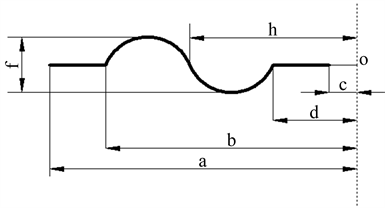Figure 1. Simplified diagram diaphragm’s model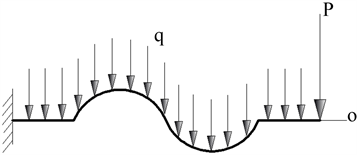Figure 2. Mechanical model of diaphragm

$\begin{array}{l}{w}_{01}=-f\frac{{\left[r-\left(d+3R\right)\right]}^{2}}{{R}^{2}},\frac{\text{d}{w}_{01}}{\text{d}r}=-f\frac{2\left[r-\left(d+3R\right)\right]}{{R}^{2}}\\ {w}_{02}=f\frac{{\left[r-\left(d+R\right)\right]}^{2}}{{R}^{2}},\frac{\text{d}{w}_{02}}{\text{d}r}=f\frac{2\left[r-\left(d+R\right)\right]}{{R}^{2}}\end{array}$

S型波纹管膜片在静态载荷下的基本方程组：

$D\frac{1}{r}\frac{\text{d}}{\text{d}r}r\frac{\text{d}}{\text{d}r}\frac{1}{r}\frac{\text{d}}{\text{d}r}r\frac{\text{d}{w}_{d}}{\text{d}r}={q}_{j}+\frac{1}{r}\frac{\text{d}}{\text{d}r}\left[r{N}_{rj}\left(\frac{\text{d}{w}_{j}}{\text{d}r}+{\lambda }_{1}\frac{\text{d}{w}_{0}}{\text{d}r}+{\lambda }_{2}\frac{\text{d}{w}_{0}}{\text{d}r}\right)\right]$ (1)

$\frac{1}{Eh}r\frac{\text{d}}{\text{d}r}\frac{1}{r}\frac{\text{d}}{\text{d}r}\left({r}^{2}{N}_{rj}\right)=-\frac{1}{2}\left(\frac{\text{d}{w}_{j}}{\text{d}r}+{\lambda }_{1}\frac{\text{d}{w}_{0}}{\text{d}r}+{\lambda }_{2}\frac{\text{d}{w}_{0}}{\text{d}r}\right)\frac{\text{d}{w}_{d}}{\text{d}r}$ (2)

$\begin{array}{l}D\frac{1}{r}\frac{\text{d}}{\text{d}r}r\frac{\text{d}}{\text{d}r}\frac{1}{r}\frac{\text{d}}{\text{d}r}r\frac{\text{d}{w}_{d}}{\text{d}r}\\ ={q}_{d}+\frac{1}{r}\frac{\text{d}}{\text{d}r}\left[r{N}_{rj}\frac{\text{d}{w}_{d}}{\text{d}r}+r{N}_{rd}\left(\frac{\text{d}{w}_{j}}{\text{d}r}+\frac{\text{d}{w}_{d}}{\text{d}r}+{\lambda }_{1}\frac{\text{d}{w}_{0}}{\text{d}r}+{\lambda }_{2}\frac{\text{d}{w}_{0}}{\text{d}r}\right)\right]-{c}^{\prime }\frac{d{w}_{d}}{dt}-\gamma \frac{{\text{d}}^{2}{w}_{d}}{\text{d}{t}^{2}}\end{array}$ (3)

$\frac{1}{Eh}r\frac{\text{d}}{\text{d}r}\frac{1}{r}\frac{\text{d}}{\text{d}r}\left({r}^{2}{N}_{rd}\right)=-\frac{1}{2}\left(\frac{\text{d}{w}_{d}}{\text{d}r}+\frac{\text{d}{w}_{j}}{\text{d}r}+{\lambda }_{1}\frac{\text{d}{w}_{0}}{\text{d}r}+{\lambda }_{2}\frac{\text{d}{w}_{0}}{\text{d}r}\right)\frac{\text{d}{w}_{d}}{\text{d}r}$ (4)

${\lambda }_{1}$ ”和“ ${\lambda }_{2}$ ”为引入的两个关于a的阶梯函数，分别为：

${\lambda }_{1},{\lambda }_{2}$ 取值：

${\lambda }_{1}=\left\{\begin{array}{l}1,\frac{c}{a}\le x\le \frac{d}{a}\\ 0,其他\end{array}$${\lambda }_{2}=\left\{\begin{array}{l}-1,\frac{h}{a}\le x\le \frac{b}{a}\\ 1,\frac{d}{a}\le x\le \frac{h}{a}\\ 0,其他\end{array}$

E代表杨氏模量， $D=\frac{E{h}^{3}}{12\left(1-{\mu }^{2}\right)}$${c}^{\prime }\text{ }$ 代表S型波纹管膜片的阻尼系数， ${w}_{0}$ 代表初挠度， $\gamma$ 代表S

$\begin{array}{l}{\int }_{{t}_{1}}^{{t}_{2}}{\int }_{c}^{a}\left\{D\frac{1}{r}\frac{\partial }{\partial r}r\frac{\partial }{\partial r}\frac{1}{r}\frac{\partial }{\partial r}r\frac{\partial }{\partial r}\left({w}_{d}\right)-{q}_{d}-\frac{1}{r}\frac{\partial }{\partial r}\left[r{N}_{rj}\frac{\partial {w}_{d}}{\partial r}+r{N}_{rd}\left(\frac{\partial {w}_{j}}{\partial r}+\frac{\partial {w}_{d}}{\partial r}+{\lambda }_{1}\frac{\partial {w}_{0}}{\partial r}+{\lambda }_{2}\frac{\partial {w}_{0}}{\partial r}\right)\right]\\ -\frac{P}{2\pi }+c\frac{\partial {w}_{d}}{\partial t}+\gamma \frac{{\partial }^{2}{w}_{d}}{\partial {t}^{2}}\right\}r\delta {w}_{d}\text{d}r\text{d}t=0\end{array}$ (5)

$\frac{1}{Eh}r\frac{\partial }{\partial r}\frac{1}{r}\frac{\partial }{\partial r}\left({r}^{2}{N}_{rd}\right)=-\left[\frac{1}{2}{\left(\frac{\partial {w}_{d}}{\partial r}\right)}^{2}+\frac{\partial {w}_{j}}{\partial r}\frac{\partial {w}_{d}}{\partial r}+{\lambda }_{1}\frac{\partial {w}_{d}}{\partial r}\frac{\partial {w}_{0}}{\partial r}+{\lambda }_{2}\frac{\partial {w}_{d}}{\partial r}\frac{\partial {w}_{0}}{\partial r}\right]$ (6)

$r=a,\text{\hspace{0.17em}}{w}_{d\left(1\right)}=0,\text{\hspace{0.17em}}\frac{\text{d}{w}_{d\left(1\right)}}{\text{d}r}=0,\text{\hspace{0.17em}}\frac{\text{d}}{\text{d}r}\left(r{N}_{r\left(1\right)}\right)-\mu {N}_{r\left(1\right)}=0$ (7)

$r=c,\text{\hspace{0.17em}}\frac{{\text{d}}^{2}{w}_{d\left(4\right)}}{\text{d}{r}^{2}}+\frac{\mu }{r}\frac{\text{d}{w}_{d\left(4\right)}}{\text{d}r}=0,\text{\hspace{0.17em}}{N}_{r\left(4\right)}=0$ (8)

$r=b,\text{\hspace{0.17em}}{w}_{d\left(1\right)}={w}_{d\left(2\right)},\text{\hspace{0.17em}}\frac{\text{d}{w}_{d\left(1\right)}}{\text{d}r}=\frac{\text{d}{w}_{d\left(2\right)}}{\text{d}r},\text{\hspace{0.17em}}{M}_{r\left(1\right)}={M}_{r\left(2\right)},\text{\hspace{0.17em}}{N}_{r\left(1\right)}={N}_{r\left(2\right)},\text{\hspace{0.17em}}{N}_{\theta \left(1\right)}={N}_{\theta \left(2\right)}$ (9)

$r=h,\text{\hspace{0.17em}}{w}_{d\left(2\right)}={w}_{d\left(3\right)},\text{\hspace{0.17em}}\frac{\text{d}{w}_{d\left(2\right)}}{\text{d}r}=\frac{\text{d}{w}_{d\left(3\right)}}{\text{d}r},\text{\hspace{0.17em}}{M}_{r\left(2\right)}={M}_{r\left(3\right)},\text{\hspace{0.17em}}{N}_{r\left(2\right)}={N}_{r\left(3\right)},\text{\hspace{0.17em}}{N}_{\theta \left(2\right)}={N}_{\theta \left(3\right)}$ (10)

$r=d,\text{\hspace{0.17em}}{w}_{d\left(3\right)}={w}_{d\left(4\right)},\text{\hspace{0.17em}}\frac{\text{d}{w}_{d\left(3\right)}}{\text{d}r}=\frac{\text{d}{w}_{d\left(4\right)}}{\text{d}r},\text{\hspace{0.17em}}{M}_{r\left(3\right)}={M}_{r\left(4\right)},\text{\hspace{0.17em}}{N}_{r\left(3\right)}={N}_{r\left(4\right)},\text{\hspace{0.17em}}{N}_{\theta \left(3\right)}={N}_{\theta \left(4\right)}$ (11)

2.2. 基本方程和边界条件的无量纲化

$\begin{array}{l}x=\frac{r}{a},\text{\hspace{0.17em}}W=\frac{w}{h},\text{\hspace{0.17em}}S=\frac{12a\left(1-{\mu }^{2}\right)r{N}_{r}a}{E{h}^{3}},\text{\hspace{0.17em}}\beta Q=\frac{12{a}^{4}\left(1-{\mu }^{2}\right)q}{E{h}^{4}},\text{\hspace{0.17em}}k=f\frac{2{a}^{2}}{{R}^{2}h},\text{\hspace{0.17em}}{\varphi }_{1}=f\frac{2a\left(d+3R\right)}{{R}^{2}h},\\ {\varphi }_{2}=-f\frac{2a\left(d+R\right)}{{R}^{2}h},\text{\hspace{0.17em}}{c}^{″}=\frac{12{a}^{4}\left(1-{\mu }^{2}\right)}{E{h}^{3}}{c}^{\prime },\text{\hspace{0.17em}}{\gamma }_{1}=\frac{12{a}^{4}\left(1-{\mu }^{2}\right)}{E{h}^{3}}\gamma ,\text{\hspace{0.17em}}\alpha =6\left(1-{\mu }^{2}\right)\end{array}$

$\begin{array}{l}{\int }_{0}^{2\pi }{\int }_{\frac{c}{a}}^{1}\left\{{L}_{1}\left({W}_{d}\right)-Q-\frac{1}{x}\frac{\partial }{\partial x}\left[{S}_{j}\frac{\partial {W}_{d}}{\partial x}+{S}_{d}\left(\frac{\partial {W}_{j}}{\partial x}+\frac{\partial {W}_{d}}{\partial x}+{\lambda }_{1}\left({\varphi }_{1}-kx\right)+{\lambda }_{2}\left(kx-{\varphi }_{2}\right)\right)\right]\\ \text{ }+{c}^{″}\frac{\partial {W}_{d}}{\partial \tau }+{\gamma }_{1}\frac{{\partial }^{2}{W}_{d}}{\partial {\tau }^{2}}\right\}x{W}_{d}\text{d}x\text{d}\tau =0\end{array}$ (12)

${L}_{2}\left(x{S}_{d}\right)=-\left[\alpha {\left(\frac{\partial {W}_{d}}{\partial x}\right)}^{2}+2\alpha \frac{\partial {W}_{j}}{\partial x}\frac{\partial {W}_{d}}{\partial x}+2\alpha \frac{\partial {W}_{d}}{\partial x}\left({\lambda }_{1}\left({\varphi }_{1}-kx\right)+{\lambda }_{2}\left(kx-{\varphi }_{2}\right)\right)\right]$ (13)

$x=1,\text{\hspace{0.17em}}{W}_{\text{d(1)}}=0,\text{\hspace{0.17em}}\frac{\text{d}{W}_{d\left(1\right)}}{\text{d}x}=0,\text{\hspace{0.17em}}\frac{\text{d}{S}_{d\left(1\right)}}{\text{d}x}-\mu {S}_{d\left(1\right)}=0$ (14)

$x=\frac{c}{a},\text{\hspace{0.17em}}\frac{{\text{d}}^{2}{W}_{d\left(4\right)}}{\text{d}{x}^{2}}+\frac{\mu }{x}\frac{\text{d}{W}_{d\left(4\right)}}{\text{d}x}=0,\text{\hspace{0.17em}}{S}_{d\left(4\right)}=0$ (15)

$\begin{array}{l}x=\frac{b}{a},\text{\hspace{0.17em}}{W}_{d\left(1\right)}={W}_{d\left(2\right)},\text{\hspace{0.17em}}\frac{{\text{d}}^{2}{W}_{d\left(1\right)}}{\text{d}{x}^{2}}+\frac{\mu }{x}\frac{\text{d}{W}_{d\left(1\right)}}{\text{d}x}=\frac{{\text{d}}^{2}{W}_{d\left(2\right)}}{\text{d}{x}^{2}}+\frac{\mu }{x}\frac{\text{d}{W}_{d\left(2\right)}}{\text{d}x},\\ \frac{\text{d}{W}_{d\left(1\right)}}{\text{d}x}=\frac{\text{d}{W}_{d\left(2\right)}}{\text{d}x},\text{\hspace{0.17em}}{S}_{d\left(1\right)}={S}_{d\left(2\right)},\text{\hspace{0.17em}}\frac{\text{d}}{\text{d}x}\left(x{S}_{d\left(1\right)}\right)=\frac{\text{d}}{\text{d}x}\left(x{S}_{d\left(2\right)}\right)\end{array}$ (16)

$\begin{array}{l}x=\frac{h}{a},\text{\hspace{0.17em}}{W}_{2\left(2\right)}={W}_{d\left(3\right)},\text{\hspace{0.17em}}\frac{{\text{d}}^{2}{W}_{d\left(2\right)}}{\text{d}{x}^{2}}+\frac{\mu }{x}\frac{\text{d}{W}_{d\left(2\right)}}{\text{d}x}=\frac{{\text{d}}^{2}{W}_{d\left(3\right)}}{\text{d}{x}^{2}}+\frac{\mu }{x}\frac{\text{d}{W}_{d\left(3\right)}}{\text{d}x},\\ \frac{\text{d}{W}_{d\left(2\right)}}{\text{d}x}=\frac{d{W}_{d\left(2\right)}}{\text{d}x},\text{\hspace{0.17em}}{S}_{d\left(2\right)}={S}_{d\left(3\right)},\text{\hspace{0.17em}}\frac{\text{d}}{\text{d}x}\left(x{S}_{d\left(2\right)}\right)=\frac{\text{d}}{\text{d}x}\left(x{S}_{d\left(3\right)}\right)\end{array}$ (17)

$\begin{array}{l}x=\frac{d}{a},\text{\hspace{0.17em}}{W}_{d\left(3\right)}={W}_{d\left(4\right)},\text{\hspace{0.17em}}\frac{{\text{d}}^{2}{W}_{d\left(3\right)}}{\text{d}{x}^{2}}+\frac{\mu }{x}\frac{\text{d}{W}_{d\left(3\right)}}{\text{d}x}=\frac{{\text{d}}^{2}{W}_{d\left(4\right)}}{\text{d}{x}^{2}}+\frac{\mu }{x}\frac{\text{d}{W}_{d\left(4\right)}}{\text{d}x},\\ \frac{\text{d}{W}_{d\left(3\right)}}{\text{d}x}=\frac{\text{d}{W}_{d\left(4\right)}}{\text{d}x},\text{\hspace{0.17em}}{S}_{d\left(3\right)}={S}_{d\left(4\right)},\text{\hspace{0.17em}}\frac{\text{d}}{\text{d}x}\left(x{S}_{d\left(3\right)}\right)=\frac{\text{d}}{\text{d}x}\left(x{S}_{d\left(4\right)}\right)\end{array}$ (18)

3. 受迫振动方程

$\begin{array}{c}{S}_{j\left(1\right)}=\frac{1}{x}\left(-\frac{1}{12288}a{Q}_{1}^{2}{x}^{8}-\frac{1}{384}a{Q}_{1}{c}_{14}{x}^{6}-\frac{1}{64}a{x}^{4}{Q}_{1}{c}_{15}-\frac{1}{32}a{x}^{4}{c}_{14}^{2}-\frac{1}{2}a{c}_{14}{c}_{15}\mathrm{ln}\left(x\right){x}^{2}\\ \text{\hspace{0.17em}}+\frac{1}{4}a{c}_{14}{c}_{15}{x}^{2}+\frac{1}{2}a{c}_{15}^{2}\mathrm{ln}\left(x\right)\right)+\frac{1}{2}{c}_{16}x+\frac{{c}_{17}}{x}\end{array}$ (19)

$\begin{array}{c}{S}_{j\left(2\right)}=\frac{1}{x}\left(-\frac{1}{12288}a{Q}_{1}^{2}{x}^{8}+\frac{1}{192}a{x}^{6}k{Q}_{1}-\frac{1}{384}a{Q}_{1}{n}_{14}{x}^{6}-\frac{1}{120}a{Q}_{1}{\varphi }_{1}{x}^{5}-\frac{1}{64}a{x}^{4}{Q}_{1}{n}_{15}\\ \text{\hspace{0.17em}}+\frac{1}{8}a{x}^{4}k{n}_{14}-\frac{1}{32}a{x}^{4}{n}_{14}^{2}+a{n}_{15}\mathrm{ln}\left(x\right)k{x}^{2}-\frac{1}{2}a{n}_{15}k{x}^{2}-\frac{1}{2}a{n}_{14}{n}_{15}\mathrm{ln}\left(x\right){x}^{2}\\ \text{\hspace{0.17em}}+\frac{1}{4}a{n}_{14}{n}_{15}{x}^{2}-\frac{1}{3}a{n}_{14}{\varphi }_{1}{x}^{3}+2a{n}_{15}{\varphi }_{1}x+\frac{1}{2}a{n}_{15}^{2}\mathrm{ln}\left(x\right)\right)+\frac{1}{2}{n}_{16}x+\frac{{n}_{17}}{x}\end{array}$ (20)

$\begin{array}{c}{S}_{j\left(3\right)}=\frac{1}{x}\left(-\frac{1}{12288}a{Q}_{1}^{2}{x}^{8}-\frac{1}{192}a{x}^{6}k{Q}_{1}-\frac{1}{384}a{Q}_{1}{m}_{14}{x}^{6}+\frac{1}{120}a{Q}_{1}{\varphi }_{1}{x}^{5}-\frac{1}{64}a{x}^{4}{Q}_{1}{m}_{15}\\ \text{\hspace{0.17em}}-\frac{1}{8}a{x}^{4}k{m}_{14}-\frac{1}{32}a{x}^{4}{m}_{14}^{2}-a{m}_{15}\mathrm{ln}\left(x\right)k{x}^{2}+\frac{1}{2}a{m}_{15}k{x}^{2}-\frac{1}{2}a{m}_{14}{m}_{15}\mathrm{ln}\left(x\right){x}^{2}\\ \text{\hspace{0.17em}}+\frac{1}{4}a{m}_{14}{m}_{15}{x}^{2}+\frac{1}{3}a{m}_{14}{\varphi }_{2}{x}^{3}-2a{m}_{15}{\varphi }_{1}x+\frac{1}{2}a{m}_{15}^{2}\mathrm{ln}\left(x\right)\right)+\frac{1}{2}{m}_{16}x+\frac{{m}_{17}}{x}\end{array}$ (21)

$\begin{array}{c}{S}_{j\left(4\right)}=\frac{1}{x}\left(-\frac{1}{12288}a{Q}_{1}^{2}{x}^{8}-\frac{1}{384}a{Q}_{1}{h}_{14}{x}^{6}-\frac{1}{64}a{x}^{4}{Q}_{1}{h}_{15}-\frac{1}{32}a{x}^{4}{h}_{14}^{2}-\frac{1}{2}a{h}_{14}{h}_{15}\mathrm{ln}\left(x\right){x}^{2}\\ \text{\hspace{0.17em}}+\frac{1}{4}a{h}_{14}{h}_{15}{x}^{2}+\frac{1}{2}a{h}_{15}^{2}\mathrm{ln}\left(x\right)\right)+\frac{1}{2}{h}_{16}x+\frac{{h}_{17}}{x}\end{array}$ (22)

${W}_{d}=\left(\frac{1}{2}{x}^{4}+{h}_{21}{x}^{2}+{h}_{22}\mathrm{ln}x+{h}_{23}\right)f\left(t\right)$ (23)

$\frac{{\text{d}}^{2}f\left(t\right)}{\text{d}{t}^{2}}+{\phi }_{0}\frac{\text{d}f\left(t\right)}{\text{d}t}+{\phi }_{1}f\left(t\right)+{\phi }_{2}{f}^{2}\left(t\right)+{\phi }_{3}{f}^{3}\left(t\right)=g\mathrm{cos}\left(\Omega t\right)$ (24)

${\phi }_{1}={\omega }^{2}$，取 $t=\omega \tau$$f\left(t\right)=\frac{\omega }{\sqrt{{\phi }_{3}}}\eta \left(\tau \right)$

$\frac{{\text{d}}^{2}\eta \left(\tau \right)}{\text{d}{\tau }^{2}}+{\alpha }^{\prime }\frac{\text{d}\eta \left(\tau \right)}{\text{d}\tau }+\eta \left(\tau \right)-{\chi }^{\prime }{\eta }^{2}\left(\tau \right)+{\eta }^{3}\left(\tau \right)={g}^{\prime }\mathrm{cos}\left(\frac{\Omega }{\omega }\tau \right)$ (25)

4. 对自由振动方程求解

$\left\{\begin{array}{l}{{\eta }^{\prime }}_{1}\left(\tau \right)={\eta }_{2}\left(\tau \right)\\ {{\eta }^{\prime }}_{2}\left(\tau \right)=-{\alpha }^{\prime }{\eta }_{1}\left(\tau \right)-{\eta }^{3}\left(\tau \right)+{\chi }^{\prime }{\eta }_{1}^{2}\left(\tau \right)-{\eta }_{1}^{3}\left(\tau \right)+{g}^{\prime }\mathrm{cos}\left(\frac{\Omega }{\omega }\tau \right)\end{array}$ (26)

$\left\{\begin{array}{l}{{\eta }^{\prime }}_{1}\left(\tau \right)={\eta }_{2}\left(\tau \right)\\ {{\eta }^{\prime }}_{2}\left(\tau \right)=-{\eta }^{3}\left(\tau \right)+{\chi }^{\prime }{\eta }_{1}^{2}\left(\tau \right)-{\eta }_{1}^{3}\left(\tau \right)\end{array}$ (27)

$H\left({\eta }_{1}\left(\tau \right),{\eta }_{2}\left(\tau \right)\right)=\frac{{\left({{\eta }^{\prime }}_{1}\left(\tau \right)\right)}^{2}}{2}+\frac{{\eta }_{1}^{2}\left(\tau \right)}{2}-\frac{{\chi }^{\prime }}{3}{\eta }_{1}^{3}\left(\tau \right)+\frac{1}{4}{\eta }_{1}^{4}\left(\tau \right)$ (28)

${\eta }_{2}^{2}={\left({{\eta }^{\prime }}_{1}\right)}^{2}=-{\eta }_{1}^{2}+\frac{2}{3}{\chi }^{\prime }{\eta }_{1}^{3}-\frac{1}{2}{\eta }_{1}^{4}$ (29)

$\frac{\text{d}{\eta }_{1}\left(\tau \right)}{\text{d}\tau }=±{\eta }_{1}\sqrt{-\frac{{\eta }_{1}^{2}}{2}+\frac{2}{3}{\chi }^{\prime }{\eta }_{1}-1}$，则 $\tau =±\left(\mathrm{arcsin}\frac{\frac{2}{3}{\chi }^{\prime }{\eta }_{1}-2}{|{\eta }_{1}|\sqrt{\frac{2}{3}{\chi }^{\prime }}-2}\right)$

$u=\frac{2}{3}{\chi }^{\prime }$$v=\sqrt{{\left(\frac{2}{3}{\chi }^{\prime }\right)}^{2}-2}=\sqrt{{u}^{2}-2}$，则 $\tau =±\left(\mathrm{arcsin}\frac{u{\eta }_{1}-2}{v{\eta }_{1}}+C\right)$

${\eta }_{1}\left(\tau \right)=\frac{2}{u±v\mathrm{sin}\tau }$${\eta }_{2}\left(\tau \right)=\frac{\mp 2v\mathrm{cos}\tau }{{\left(u±v\mathrm{sin}\tau \right)}^{2}}$

$\left({\eta }_{1}\left(\tau \right),{\eta }_{2}\left(\tau \right)\right)=\left(\frac{2}{u+v\mathrm{sin}\tau },\frac{-2v\mathrm{cos}\tau }{{\left(u+v\mathrm{sin}\tau \right)}^{2}}\right)$

$\left({\eta }_{1}\left(\tau \right),{\eta }_{2}\left(\tau \right)\right)=\left(\frac{2}{u-v\mathrm{sin}\tau },\frac{2v\mathrm{cos}\tau }{{\left(u-v\mathrm{sin}\tau \right)}^{2}}\right)$

5. 研究平衡点处的稳定性

$\left\{\begin{array}{l}{{\eta }^{\prime }}_{1}\left(\tau \right)={\eta }_{2}\left(\tau \right)\\ {{\eta }^{\prime }}_{2}\left(\tau \right)=-{\alpha }^{\prime }{\eta }_{2}\left(\tau \right)-{\eta }_{1}\left(\tau \right)+{\chi }^{\prime }{\eta }_{1}^{2}\left(\tau \right)-{\eta }_{1}^{3}\left(\tau \right)\end{array}$ (30)

$\left\{\begin{array}{l}{f}_{1}\left({\eta }_{1},{\eta }_{2}\right)={\eta }_{2}\left(\tau \right)\\ {f}_{2}\left({\eta }_{1},{\eta }_{2}\right)=-{\alpha }^{\prime }{\eta }_{2}\left(\tau \right)-{\eta }_{1}\left(\tau \right)+{\chi }^{\prime }{\eta }_{1}^{2}\left(\tau \right)-{\eta }_{1}^{3}\left(\tau \right)\end{array}$

Jacobi矩阵是：

$\left[\begin{array}{cc}\frac{\partial {f}_{1}}{\partial {\eta }_{1}}& \frac{\partial {f}_{1}}{\partial {\eta }_{2}}\\ \frac{\partial {f}_{2}}{\partial {\eta }_{1}}& \frac{\partial {f}_{2}}{\partial {\eta }_{2}}\end{array}\right]=\left[\begin{array}{cc}0& 1\\ -1+2{\chi }^{\prime }{\eta }_{1}-3{\eta }_{1}^{2}& -{\alpha }^{\prime }\end{array}\right]$

1) 在平衡点是 $\left(0,0\right)$ 情况，Jacobi矩阵为 $\left[\begin{array}{cc}0& 1\\ -1& -{\alpha }^{\prime }\end{array}\right]$

$0<{\alpha }^{\prime }<2$ 的情况下， ${\delta }_{1,2}=\frac{-{\alpha }^{\prime }}{2}±i\sqrt{1-{\left(\frac{{\alpha }^{\prime }}{2}\right)}^{2}}$ 是不等的复数，复平面上有稳定交点，图3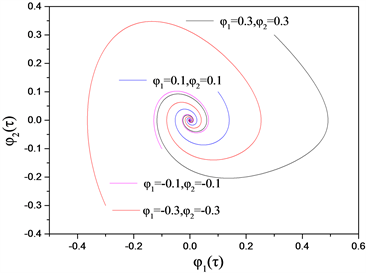${\alpha }^{\prime }=0.5$ ${\chi }^{\prime }=2$

Figure 3. Phase diagram ( $0<{\alpha }^{\prime }<2$ )

${\alpha }^{\prime }=2$ 的情况下， ${\delta }_{1,2}=-1$ 是相等负数，平衡点是临界结点，图4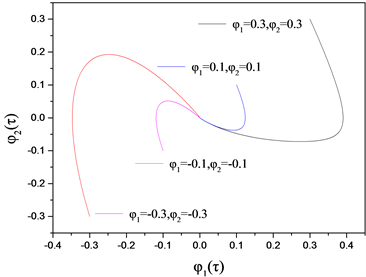${\alpha }^{\prime }=2$ ${\chi }^{\prime }=2$

Figure 4. Phase diagram ( ${\alpha }^{\prime }=2$ )

${\alpha }^{\prime }>2$ 的情况下， ${\delta }_{1,2}=-\frac{{\alpha }^{\prime }}{2}±\sqrt{{\left(\frac{{\alpha }^{\prime }}{2}\right)}^{2}-1}$ 是不等负数，平衡点是稳定结点，图5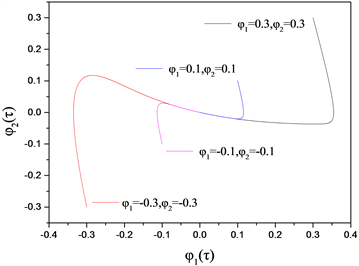${\alpha }^{\prime }=4$ ${\chi }^{\prime }=2$

Figure 5. Phase diagram ( ${\alpha }^{\prime }>2$ )

${\alpha }^{\prime }=0$ 的情况下， ${\delta }_{1,2}=±i$ 是纯虚数，求得曲线是极限环，图6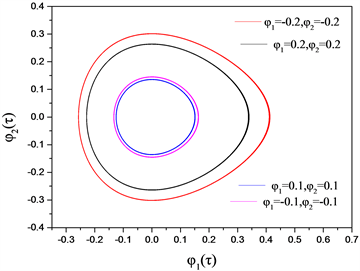${\chi }^{\prime }=2$

Figure 6. Phase diagram ( ${\alpha }^{\prime }=0$ )

2) 在平衡点 $\left(\frac{{\chi }^{\prime }}{2}+\sqrt{{\left(\frac{{\chi }^{\prime }}{2}\right)}^{2}-1},0\right)$

Jacobi矩阵是 $\left[\begin{array}{cc}0& 1\\ \frac{4-{\left({\chi }^{\prime }\right)}^{2}-{\chi }^{\prime }\sqrt{{\left({\chi }^{\prime }\right)}^{2}-4}}{2}& -{\alpha }^{\prime }\end{array}\right]$，特征方程是 $|\begin{array}{l}\text{}0-\delta \text{}1\text{}\\ 2-\frac{{\left({\chi }^{\prime }\right)}^{2}}{2}-\frac{{\chi }^{\prime }}{2}\sqrt{{\left({\chi }^{\prime }\right)}^{2}-4}\text{}-{\alpha }^{\prime }-\delta \end{array}|=0$，解得 ${\delta }_{1,2}=\frac{-{\alpha }^{\prime }±\sqrt{{\left({\alpha }^{\prime }\right)}^{2}+\left(8-2{\left({\chi }^{\prime }\right)}^{2}-2{\chi }^{\prime }\sqrt{{\left({\chi }^{\prime }\right)}^{2}-4}\right)}}{2}$

$0<{\alpha }^{\prime }<\sqrt{-8+2{\left({\chi }^{\prime }\right)}^{2}-2{\chi }^{\prime }\sqrt{{\left({\chi }^{\prime }\right)}^{2}-4}}$ 情况下， ${\delta }_{1,2}=\frac{-{\alpha }^{\prime }}{2}±\frac{i}{2}\sqrt{-{\left({\alpha }^{\prime }\right)}^{2}-8+2{\left({\chi }^{\prime }\right)}^{2}+2{\chi }^{\prime }\sqrt{{\left({\chi }^{\prime }\right)}^{2}-4}}$ 是不等复数，复平面有稳定交点；

${\alpha }^{\prime }=\sqrt{-8+2{\left({\chi }^{\prime }\right)}^{2}+2{\chi }^{\prime }\sqrt{{\left({\chi }^{\prime }\right)}^{2}-4}}$ 情况下， ${\delta }_{1,2}=-\frac{{\alpha }^{\prime }}{2}$ 是相等负数， $\left(\frac{{\chi }^{\prime }}{2}+\sqrt{{\left(\frac{{\chi }^{\prime }}{2}\right)}^{2}-1},0\right)$ 是临界结点；

${\alpha }^{\prime }>\sqrt{-8+2{\left({\chi }^{\prime }\right)}^{2}+2{\chi }^{\prime }\sqrt{{\left({\chi }^{\prime }\right)}^{2}-4}}$ 情况下， ${\delta }_{1,2}=\frac{-{\alpha }^{\prime }±\sqrt{{\left({\alpha }^{\prime }\right)}^{2}-\left(8-2{\left({\chi }^{\prime }\right)}^{2}+2{\chi }^{\prime }\sqrt{{\left({\chi }^{\prime }\right)}^{2}-4}\right)}}{2}$ 是不等负数， $\left(\frac{{\chi }^{\prime }}{2}+\sqrt{{\left(\frac{{\chi }^{\prime }}{2}\right)}^{2}-1},0\right)$ 是稳定结点；

${\alpha }^{\prime }=0$ 情况下，特征根 ${\delta }_{1,2}=±i\sqrt{-8+2{\left({\chi }^{\prime }\right)}^{2}+2{\chi }^{\prime }\sqrt{{\left({\chi }^{\prime }\right)}^{2}-4}}$ 是纯虚数，有Hopf分岔。

3) 在平衡点 $\left(\frac{{\chi }^{\prime }}{2}-\sqrt{{\left(\frac{{\chi }^{\prime }}{2}\right)}^{2}-1},0\right)$

Jacobi矩阵 $\left[\begin{array}{cc}0& 1\\ \frac{4-{\left({\chi }^{\prime }\right)}^{2}+{\chi }^{\prime }\sqrt{{\left({\chi }^{\prime }\right)}^{2}-4}}{2}& -{\alpha }^{\prime }\end{array}\right]$$\left(\frac{{\chi }^{\prime }}{2}-\sqrt{{\left(\frac{{\chi }^{\prime }}{2}\right)}^{2}-1},0\right)$ 处的特征根是 ${\delta }_{1,2}=\frac{-{\alpha }^{\prime }±\sqrt{{\left({\alpha }^{\prime }\right)}^{2}-8+2{\left({\chi }^{\prime }\right)}^{2}-2{\chi }^{\prime }\sqrt{{\left({\chi }^{\prime }\right)}^{2}-4}}}{2}$${\delta }_{1,2}$ 是不等负数，平衡点是稳定结点。

6. 小结

1) S型波纹管非线性力学自由振动方程，准确解

$\left({\eta }_{1}\left(\tau \right),{\eta }_{2}\left(\tau \right)\right)=\left(\frac{2}{u+v\mathrm{sin}\tau },\frac{-2v\mathrm{cos}\tau }{{\left(u+v\mathrm{sin}\tau \right)}^{2}}\right)$

$\left({\eta }_{1}\left(\tau \right),{\eta }_{2}\left(\tau \right)\right)=\left(\frac{2}{u-v\mathrm{sin}\tau },\frac{2v\mathrm{cos}\tau }{{\left(u-v\mathrm{sin}\tau \right)}^{2}}\right)$

2) S型波纹管在动静载荷下，平衡点 $\left(0,0\right)$ 处，在 $0<{\alpha }^{\prime }<2$ 的情况下，根是不等复数，有稳定的交点；在 ${\alpha }^{\prime }=2$ 的情况下，根是相等负数，平衡点是临界点；在 ${\alpha }^{\prime }>2$ 的情况，根为不等负数，平衡点为稳定结点；在 ${\alpha }^{\prime }=0$ 的情况下，发生Hopf分岔。

NOTES

*通讯作者。

  张静, 丁雪兴, 黄义仿, 等. 干气密封焊接金属波纹管的结构动态分析[J]. 化工机械, 2010, 37(2): 183-185.  刘静, 黄卫良, 李霄, 等. 单双层U形不锈钢波纹管液压胀形变形特征对比研究[J]. 锻压技术, 2016, 41(5): 24-28.  高洪彪. 横向载荷下弯曲波纹管的变形与吸能特性[D]: [硕士学位论文]. 太原: 太原理工大学, 2016.  阿斯耶姆•肖开提, 刘焕海. 焊接金属波纹管机械密封温度场及热变形有限元分析研究[J]. 科技视界, 2016(4): 25-26.  Faraji, G., Besharati, M.K., Mosavi, M., et al. (2008) Experimental and Finite Element Analysis of Parameters in Manufacturing of Metal Bellows. The International Journal of Advanced Manufacturing Technology, 38, 641-648. https://doi.org/10.1007/s00170-007-1122-9  Kumar Krovvidi, S.C.S.P., Padmakumar, G. and Bhaduri, A.K. (2016) Design and Analysis of Bellows for High Temperature Application as Per RCC-MR. Transactions of the Indian Institute of Metals, 69, 531-535. https://doi.org/10.1007/s12666-015-0813-4  Liang, H. (1993) On Problems of U-Shaped Bellows with Non-linear Deformation of Large Axisymmetrical Deflection (II)—Counting Variation of Thickness Distribution. Applied Mathematics and Mechanics, 14, 559-564. https://doi.org/10.1007/BF02451365  Chand, S. and Garg, S.B.L. (1981) Design Formulas for Expansion Bellows. Journal of Mechanical Design, 103, 881-891. https://doi.org/10.1115/1.3255001  李树勋, 张丽芳, 吕兴, 等. 二级波纹管截止阀用波纹管结构强度及疲劳可靠性研究[J]. 流体机械, 2018, 46(11): 39-44.  宋林红, 黄乃宁, 等. 金属波纹管疲劳寿命的有限元分析[J]. 管道技术与设备, 2008(11): 16-18.  王占彬, 周浩洋, 石朝锋, 等. 一种U型波纹管强度及稳定性分析方法研究[J]. 强度与环境, 2012, 39(4): 21-26.  韩明君, 王伟兵, 李鸿瑞, 等. 单圆弧波纹管膜片的非线性大变形分析[J]. 工程力学, 2020, 37(1): 26-33.  Dinesh Babu, P., Keerthi Prasath, S., Barani Dharan, M., Venkat Raman, C., Narayanan, R. and Ganesh, K.C. (2014) Analysis of Static Mechanical Behaviour of Metal Bellows Using Finite Element Modeling. Applied Mechanics and Materials, 592-594, 996-1000. https://doi.org/10.4028/www.scientific.net/AMM.592-594.996  Belyaev, A.K., Zinovieva, T.V. and Smirnov, K.K. (2017) Theoretical and Experimental Studies of the Stress-Strain State of Expansion Bellows as Elastic Shells. St. Petersburg Polytechnical University Journal: Physics and Mathematics, 3, 7-14. https://doi.org/10.1016/j.spjpm.2017.03.003